# Delta and Wye 3-Phase Circuits

## AC Electric Circuits

• #### Question 1

Label where each of the following electrical quantities would be found in both the “Y” and “Delta” three-phase configurations:

Phase voltage
Line voltage
Phase current
Line currentIn which circuit (Y or Delta) are the phase and line currents equal? In which circuit (Y or Delta) are the phase and line voltages equal? Explain both answers, in terms that anyone with a basic knowledge of electricity could understand.

Where phase and line quantities are unequal, determine which is larger.

• #### Question 2

Explain the difference between a balanced polyphase system and an unbalanced polyphase system. What conditions typically cause a polyphase system to become unbalanced?

• #### Question 3

In a balanced Y-connected power system, calculate the phase voltage (Ephase) if the line voltage (Eline) is 480 volts.

• #### Question 4

Calculate all voltages, currents, and total power in this balanced Delta-Delta system: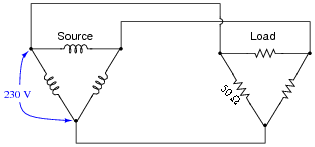Eline =
Iline =
Ephase(source) =
Iphase(source) =
Ptotal =
• #### Question 5

Calculate all voltages, currents, and total power in this balanced Y-Y system: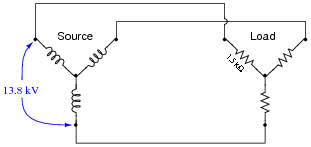Eline =
Iline =
Ephase(source) =
Iphase(source) =
Ptotal =
• #### Question 6

Calculate all voltages, currents, and total power in this balanced Delta-Y system:Eline =
Iline =
Ephase(source) =
Iphase(source) =
Ptotal =
• #### Question 7

Calculate all voltages, currents, and total power in this balanced Y-Delta system:Eline =
Iline =
Ephase(source) =
Iphase(source) =
Ptotal =
• #### Question 8

What resistor values would we have to choose in a Delta configuration to behave exactly the same as this Y-connected resistor network?• #### Question 9

What will happen in each of these systems to the phase voltages of the load, if one of the source phases fails open?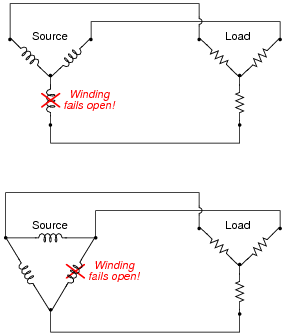• #### Question 10

A common three-phase source connection scheme is the Delta high-leg or Four-wire Delta, where each phase coil outputs 240 volts:Identify the different voltages obtained from this coil configuration, and which connection points each voltage is measured between.

• #### Question 11

Identify the primary-secondary connection configuration of these three power transformers (i.e. Y-Y, Y-Delta, Delta-Y, etc.):• #### Question 12

An electrical lineman is connecting three single-phase transformers in a Y(primary)-Y(secondary) configuration, for power service to a business. Draw the connecting wires necessary between the transformer windings, and between the transformer terminals and the lines: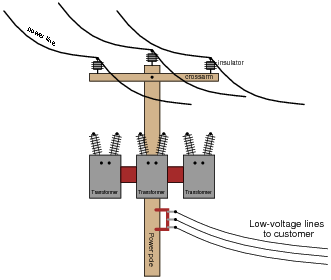Note: fuses have been omitted from this illustration, for simplicity.

• #### Question 13

Identify the primary-secondary connection configuration of these pole-mounted power transformers (i.e. Y-Y, Y-Delta, Delta-Y, etc.):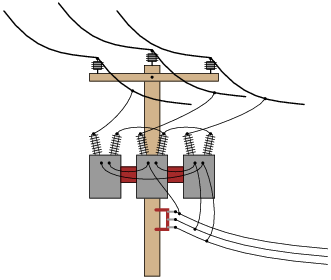• #### Question 14

Identify the primary-secondary connection configuration of these pole-mounted power transformers (i.e. Y-Y, Y-Delta, Delta-Y, etc.):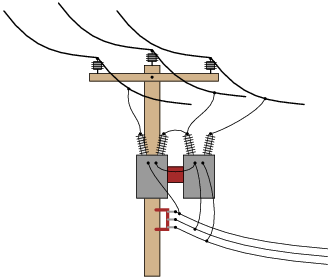• #### Question 15

One of the conductors connecting the secondary of a three-phase power distribution transformer to a large office building fails open. Upon inspection, the source of the failure is obvious: the wire overheated at a point of contact with a terminal block, until it physically separated from the terminal.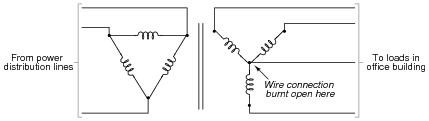What is strange, though, is that the overheated wire is the neutral conductor, not any one of the “line” conductors. Based on this observation, what do you think caused the failure?

After repairing the wire, what would you do to verify the cause of the failure?